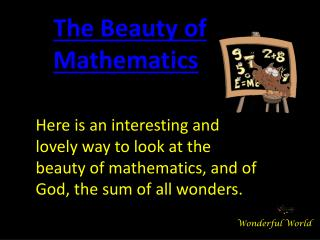# Beauty of mathematics - PowerPoint PPT PresentationDownload PresentationBeauty of mathematics

Beauty of mathematics
Download Presentation## Beauty of mathematics

- - - - - - - - - - - - - - - - - - - - - - - - - - - E N D - - - - - - - - - - - - - - - - - - - - - - - - - - -
##### Presentation Transcript

1. The Beauty of Mathematics Here is an interesting and lovely way to look at the beauty of mathematics, and of God, the sum of all wonders. Wonderful World

2. 1 x 8 + 1 = 912 x 8 + 2 = 98123 x 8 + 3 = 9871234 x 8 + 4 = 987612345 x 8 + 5 = 98765123456 x 8 + 6 = 9876541234567 x 8 + 7 = 987654312345678 x 8 + 8 = 98765432123456789 x 8 + 9 = 987654321

3. 1 x 9 + 2 = 1112 x 9 + 3 = 111123 x 9 + 4 = 11111234 x 9 + 5 = 1111112345 x 9 + 6 = 111111123456 x 9 + 7 = 11111111234567 x 9 + 8 = 1111111112345678 x 9 + 9 = 111111111123456789 x 9 +10= 1111111111

4. 9 x 9 + 7 = 8898 x 9 + 6 = 888987 x 9 + 5 = 88889876 x 9 + 4 = 8888898765 x 9 + 3 = 888888987654 x 9 + 2 = 88888889876543 x 9 + 1 = 8888888898765432 x 9 + 0 = 888888888 Brilliant, isn’t it?

5. And look at this symmetry: 1 x 1 = 111 x 11 = 121111 x 111 = 123211111 x 1111 = 123432111111 x 11111 = 123454321111111 x 111111 = 123456543211111111 x 1111111 = 123456765432111111111 x 11111111 = 123456787654321111111111 x 111111111 = 12345678987654321

6. Now, take a look at this… 101% From a strictly mathematical viewpoint: What Equals 100%? What does it mean to give MORE than 100%? Ever wonder about those people who say they are giving more than 100%? We have all been in situations where someone wants you to GIVE OVER 100% How about ACHIEVING 101%? What equals 100% in life?

7. Here’s a little mathematical formula that might help Answer these questions:

8. If: A B C D E F G H I J K L M N O P Q R S T U V W X Y Z Is represented as: 1 2 3 4 5 6 7 8 9 10 11 12 13 14 15 16 17 18 19 20 21 22 23 24 25 26.

9. If: H-A-R-D-W-O-R- K 8+1+18+4+23+15+18+11 = 98% And: K-N-O-W-L-E-D-G-E 11+14+15+23+12+5+4+7+5 = 96%

10. But: A-T-T-I-T-U-D-E 1+20+20+9+20+21+4+5 = 100% THEN, look how far the love of God will take you: L-O-V-E-O-F-G-O-D 12+15+22+5+15+6+7+15+4 = 101%

11. Therefore, one can conclude with mathematical certainty that: While Hard Work and Knowledge will get you close, and Attitude willGet you there, It’s the Love of God that will put you over the top!

12.  Have a great day… and may God bless you!